### Home > INT3 > Chapter 12 > Lesson 12.2.1 > Problem12-79

12-79.
1. Add or subtract and simplify each of the following expressions. Homework Help ✎

1.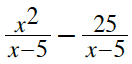2.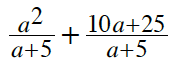3.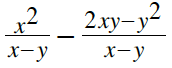4.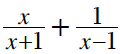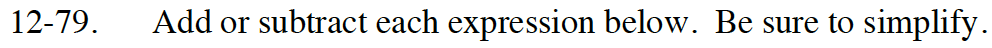Factor after adding or subtracting.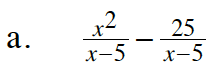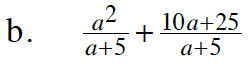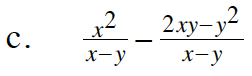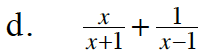In part (d), notice that the denominators are different. Find a common denominator before adding.

$\frac{x^2+1}{x^2-1}$